# How to Add the Current Month or Year in a Cell in Excel

This post will guide you how to add the current month or year into a cell or header or footer in your worksheet. How do I insert the current date or time in a cell with a formula in Excel.

## Add Current Month or Year Using Short Keys

Assuming that you want to insert current year or month into a cell or header in Excel, you can use one short cut to achieve the result. Just do the following steps:

#1 you can press Ctrl + ; keys to insert the current date in a cell.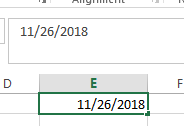#2 press one space to insert one blank character, and then press Ctrl + Shift +; to insert the current time in that cell.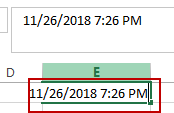## Add Current Month or Year Using Formula

You can also use a formula to insert the current year, month, day or a date or time in one cell.

Insert current Year

To insert the current year into a cell or footer or header in Excel, you can use a formula based on the YEAR function and the TODAY function. Just like this:

`=YEAR(TODAY())`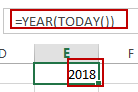Type this formula into a blank cell or footer or header in your worksheet, and then press Enter key in your keyboard.

Insert Current Month

If you want to insert the current month, you can use a formula based on the MONTH function and the TODAY function to achieve the result. Like this:

`=MONTH(TODAY())`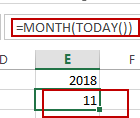Insert Current Day

To Insert the current day in a cell, you can use the below formula based on the TODAY function.

`=TODAY()`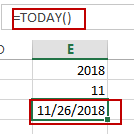Insert Current Date and Time

If you want to insert the current date and time into one cell, you can directly use the NOW function. Like this:

`=NOW()`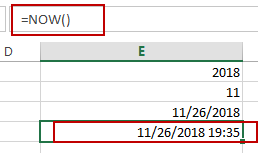### Related Functions

• Excel YEAR function
The Excel YEAR function returns a four-digit year from a given date value, the year is returned as an integer ranging from 1900 to 9999. The syntax of the YEAR function is as below:=YEAR (serial_number)…
• Excel MONTH function
The Excel MONTH function returns the month of a date represented by a serial number. And the month is an integer number from 1 to 12. The syntax of the MONTH function is as below:=MONTH (serial_number)…
• Excel TODAY function
The Excel TODAY function returns the serial number of the current date. So you can get the current system date from the TODAY function. The syntax of the TODAY function is as below:=TODAY()…
• Excel NOW function
The Excel NOW function returns the serial number of the current date and time. And it will be updated each time when your worksheet is changed or opened.The syntax of the NOW function is as below:=now()…